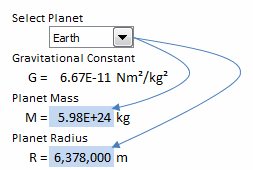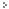If you want to know how to make excel work with tabular data in a user friendly way then our latest video tutorial is for you. The first part of the tutorial describes use of the Excel INDEX function for tabular lookups and the use of a combo box for selection. A second video covers the use of data validation boxes and the Excel MATCH function.Using Tabular Data in Excel Calculations Part 1 of 2Using Tabular Data in Excel Calculations Part 2 of 2

This is just one item in our Excel Tips series. Other subjects include:

• Using Sprites in Excel Charts - Learn how to use Excel shapes with charts to create animations.
• Easy Excel Gannt Charts - How to make Gannt charts using the Excel REPT() function.
• In Cell Charts - Make compact charts inside worksheet cells using Excel's REPT() function and Excel's conditional for...
• The Change Unit add-in for Excel - ChangeUnits is the original natural syntax unit conversion add-in for Excel. ChangeUnits understands...
• Using Excel Trendlines - Use of the LINEST function to dynamically determine equation parameters for interpolation between da...
• Reading graphs - Using Excel trendlines to generate equations from graphical or tabular data
• Matrix Operations - Learn how to work with matricies using MS Excel.
• Making great sketches with Excel.

See the full series by following this link: